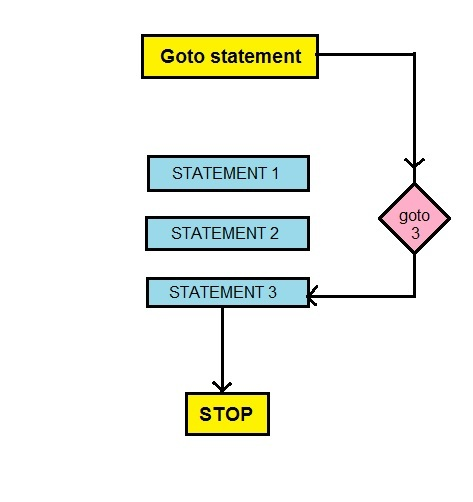# goto statement in R Programming

• Last Updated : 26 May, 2020

Goto statement in a general programming sense is a command that takes the code to the specified line or block of code provided to it. This is helpful when the need is to jump from one programming section to the other without the use of functions and without creating an abnormal shift.

Unfortunately, R doesn’t support goto but its algorithm can be easily converted to depict its application. By using following methods this can be carried out more smoothly:

• Use of if and else
• Using break, next and return

Flowchart1. Goto encountered
2. Jump to the specified line number/ name of the code block
3. Execute code

Example 1: Program to check for even and odd numbers

 `a <``-` `4``if` `((a ``%``%` `2``) ``=``=` `0``)``{ ``    ``print``(``"even"``) ``} ``else` `{``    ``print``(``"odd"``)``}`

Output:

` "even"`

Explanation:

• With goto:
1. Two blocks named EVEN and ODD
2. Evaluate for a
3. if even, goto block named EVEN
4. if odd, goto block named ODD
• Without goto:
1. Evaluate for a
2. if even, run the statement within if block
3. if odd, run the statement within else block

Example 2: Program to check for prime numbers

 `a <``-` `16``b <``-` `a``/``2``flag <``-` `0``i <``-` `2``repeat``{``    ``if` `((a ``%``%` `i)``=``=` `0``)``    ``{``        ``flag <``-` `1``        ``break` `    ``} ``}`` ` `if` `(flag ``=``=` `1``)``{``    ``print``(``"composite"``)``}``else` `{``    ``print``(``"prime"``)``}`

Output:

` "composite"`

Explanation:

• With goto :
1. This doesn’t require flag and if statement to check flag.
2. Evaluate for a
3. If a factor is found take the control to the line number that has the print statement – print(“composite).
4. If not take it to the line number that has statement – print(“prime”)
• Without goto:
1. Evaluate for a
2. If factor found, change flag
3. when the loop is complete check flag
4. Print accordingly

Note: Since R doesn’t have the concept of the goto statement, the above examples were made using simple if-else and break statements.

My Personal Notes arrow_drop_up